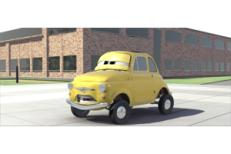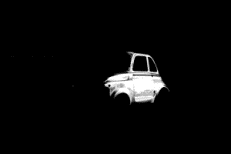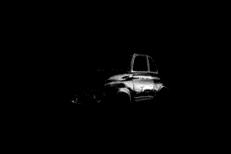IceMan Reference Guide

Region Selection

Operations in this section have to do with selecting arbitrarily shaped regions in images, a process also called image segmentation. All operations generate mattes, which are single-channel images describing region "membership". A matte pixel is 1 if the corresponding image pixel belongs to a region, and 0 if it does not. Intermediate values indicate "partial" membership and serve to soften, or feather, matte edges.

seedPoint: Location of "nucleus" (List)
startRadius: Color distance below which matte value is unity (Number)
endRadius: Color distance above which matte value is zero (Number)

Seed-filling, or region-growing, operation. Returns a matte image. Starting at a specified location, a region of contiguous pixels is computed such that the pixels satisfy a color proximity criterion. Pixels closer than startRadius generate a matte value of unity, and pixels further away than endRadius generate a matte value of zero. Intermediate values roll-off linearly. Useful values for the distance are in the 10-100 range.point := List with(608,260) result := image SeedFill(point, 10, 40))

Note: The color proximity metric used is Euclidean in the n-space that the pixels inhabit, where n is the number of channels. No guarantees of perceptual proximity are made: images must be transformed into a suitable space before attempting the fill.

seedColor: Color of pixel to be used as the "cluster" center (List)
startRadius: Color distance below which matte value is unity (Number)
endRadius: Color distance above which matte value is zero (Number)

Select all pixels in an image satisfying a certain proximity criterion. Pixels closer than startRadius generate a matte value of unity, and pixels further away than endRadius generate a matte value of zero. Intermediate values roll-off linearly. Useful values for the distance are in the 10-100 range.# Note that this operation gets non-contiguous pixels, too color := List with(0.384, 0.294, 0.0745) result := cdev ColorKey(color, 0, 15)

Note: The color proximity metric used is Euclidean in the n-space that the pixels inhabit, where n is the number of channels. No guarantees of perceptual proximity are made: images must be transformed into a suitable space before attempting the fill

x: x coord start seed fill operation
y: y coord to start seed fill operation
seedColor: Color of pixel to be used as the "cluster" center (List)
startRadius: Color distance below which matte value is unity (Number)
endRadius: Color distance above which matte value is zero (Number)

Seed-filling, or region-growing, operation. Returns a matte image. Starting at a specified location, a region of contiguous pixels is computed such that the pixels satisfy a color proximity criterion. Pixels closer than startRadius generate a matte value of unity, and pixels further away than endRadius generate a matte value of zero. Intermediate values roll-off linearly. Useful values for the distance are in the 10-100 range.

This version differs from ColorFill in that a pixel different from the one at the "nucleus" can be specified.